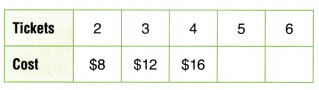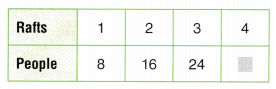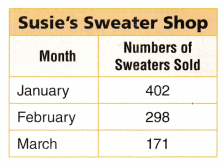Refer to our Texas Go Math Grade 3 Answer Key Pdf to score good marks in the exams. Test yourself by practicing the problems from Texas Go Math Grade 3 Unit 3 Assessment Answer Key.

Vocabulary

Choose the best term from the box to complete the sentence.

Vocabulary
expression
pattern
rule

Question 1.
An ___________ is a part of a number sentence that has numbers and operation signs but does not have an equal sign. (p. 455)
Explanation:
An expression is a part of a number sentence that has numbers and operation signs but does not have an equal sign.

Question 2.
A ___________ is an ordered set of numbers or objects in which the order helps you predict what comes next. (p. 443)
Explanation:
A pattern is an ordered set of numbers or objects in which the order helps you predict what comes next

Concepts and Skills

Write a rule for the pattern. Then write the missing number. (TEKS 3.5)

Question 3.
50, 45, 40, 35, 30, __________
Rule: _____________
Rule: subtract 5
50, 45, 40, 35, 30, 25
Explanation:
The rule is to find what comes next

Question 4.
32, 35, 38, ______, 44, 47, _________
Rule: ___________
32, 35, 38, 41, 44, 47, 50
Explanation:
The rule is to find what comes next

Find the unknown number. (TEKS 3.5.B, 3.5.D)

Question 5.
m × 5 = 30
m = _________
Explanation:
Found the unknown number with the help of inverse operations

Question 6.
48 ÷ ☐ = 6
☐ = ___________
Explanation:
Found the unknown number with the help of inverse operations

Question 7.
20 = 2 × n
n = __________
Explanation:
Found the unknown number with the help of inverse operations

Question 8.
p ÷ 8 = 4
p = _________
Explanation:
Found the unknown number with the help of inverse operations

Question 9.
7 = 63 ÷ y
y = __________
Explanation:
Found the unknown number with the help of inverse operations

Question 10.
1 × 10 = ☐
☐ = __________
Explanation:
Found the unknown number with the help of inverse operations

Describe a pattern for the table. Then complete the table. (TEKS 3.5.E)

Question 11.Explanation:
The pattern is multiples of 7

Question 12.Explanation:
multiply the question with 4

Fill in the bubble for the correct answer choice.

Question 13.
Sally has 4 comic books. Renaldo has 6 comic books. Jay has 2 times as many comic books as Sally and Renaldo combined. Which expression represents the number of comic books Jay has compared to Sally and Renaldo combined? (TEKS 3.5.C)
(A) 4 + 6
(B) 2 × 6
(C) 2 × 10
(D) 2 – 10
Explanation:
Sally has 4 comic books.
Renaldo has 6 comic books.
Jay has 2 times as many comic books as Sally and Renaldo combined.
2 x 10 = 20 expression represents the number of comic books Jay has compared to Sally and Renaldo combined

Question 14.
The camping club rents 4 rafts. How many people can 4 rafts hold? (TEKS 3.5.E)(A) 20
(B) 30
(C) 40
(D) 32
Explanation:
8 x 4 = 32
The pattern is to find the missing number which is the multiple of 8

Question 15.
There are 24 students in Mr. Smith’s class and 30 students in Mr. Becker’s class. The students sit in chairs in the gymnasium in rows with 6 chairs in each row. How many rows of chairs are there in all? (TEKS 3.5.B, 3.5.D)
(A) 54
(B) 4
(C) 9
(D) 5
Explanation:
9 x 6 = 54
There are 24 students in Mr. Smith’s class and 30 students in Mr. Becker’s class.
24 + 30 = 54

Question 16.
Use the array. Which number makes the equation true? (TEKS 3.5.B, 3.5.D)
24 ÷ 4 = ___________(A) 6
(B) 8
(C) 20
(D) 12
Explanation:
24 ÷ 4 = 8
8 x 4 = 24
This is inverse operation.

Fill in the bubble for the correct answer choice.

Use the table for 17-20. You may use models, number lines, and equations to represent and solve.Question 17.
Susie’s Sweater Shop sells sweaters online. The table ‘ shows the number of sweaters sold in three months. How many more sweaters were sold in January than in March? (TEKS 3.5.A)
(A) 231
(B) 371
(C) 331
(D) 573
Explanation:
January – march
402 – 171 = 231 more sweaters were sold in January than in March

Question 18.
How many sweaters were sold in January and February? (TEKS 3.5.A)
(A) 600
(B) 700
(C) 800
(D) 690
January + February
402 + 298 = 700 sweaters were sold in January and February

Question 19.
How many more sweaters were sold in February and March than in January? (TEKS 3.5.A)
(A) 66
(B) 167
(C) 67
(D) 871
February + march
298 + 171 =  469
469 – 402 = 67 more sweaters were sold in February and March than in January

Question 20.
Susie sold only 28 sweaters in June, 19 sweaters in July, and 11 sweaters in August. How many sweaters did she sell in June, July, and August? (TEKS 3.5.A)
(A) 57
(B) 56
(C) 48
(D) 58
28 sweaters in June, 19 sweaters in July, and 11 sweaters in August.
28 + 19 + 11 = 58

Fill in the bubble for the correct answer choice. You can use models or equations to solve.

Question 21.
Brooklyn has 10 dolphin stickers. Jorge’s stickers can be represented by 3 × 10. Which comparison statement describes the expression?
(A) Brooklyn has 10 more stickers than Jorge.
(B) Jorge has 3 times as many stickers as Brooklyn.
(C) Brooklyn has 3 more stickers than Jorge.
(D) Jorge has 3 fewer stickers than Brooklyn.
3 x 10 = 30
so, Jorge has 3 times as many stickers as Brooklyn.

Question 22.
Which of the following multiplication equations can be used to find 42 ÷ 7? (TEKS 3.5.B)
(A) 6 × 6 = 36
(B) 5 × 8 = 40
(C) 8 × 6 = 48
(D) 7 × 6 = 42
Explanation:
42 ÷ 7 = 6
7 × 6 = 42
They are inverse equations

Question 23.
Last week Arabeth read 123 pages in her book. This week she read 136 pages. There are 575 pages in the book. How many pages does she have left to read?
(A) 452
(B) 256
(C) 316
(D) Not here
Explanation:
123 + 136 = 259
575 – 259 = 316 has left to read

Question 24.
Mr. Kane keeps track of ticket sales at The Playhouse. The table shows the tickets sold for afternoon and evening shows on Friday, Saturday, and Sunday.Each show has the same number of seats available. At the Friday evening show, there were 31 empty seats. How many empty seats were there at one of the other shows? Explain how you found your answer. (TEKS 3.5.A)
Answer: 94 + 31 = 125 seats are in total
Explanation:
With the help of clue we have found that total number of seats are 125
to found the empty seats of  other shows
125 – 53 = 72

Scroll to Top